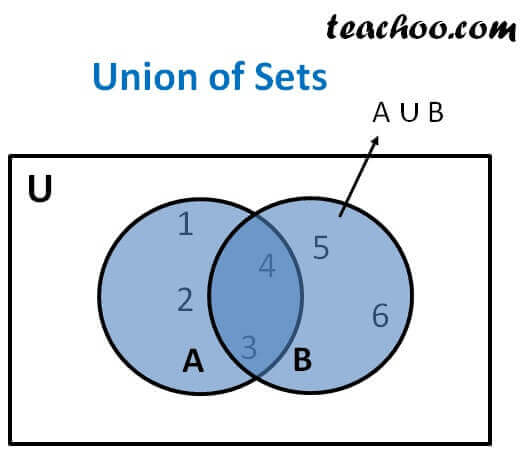Venn Diagram and Union of Set

Chapter 1 Class 11 Sets
Concept wise

Union of sets A & B has all the elements of set A and set B

It is represented by symbol ∪

Let A = {1, 2, 3, 4} , B = {3, 4, 5, 6}

A ∪ B = {1, 2, 3, 4, 5, 6}The blue region is A ∪ B

## Properties of Union

1. A ∪ B = B ∪ A (Commutative law)
2. (A ∪ B) ∪ C = A ∪ (B ∪ C) (Associative law )
3. A ∪ ∅ = A (Law of identity element, ∅ is the identity of ∪)
4. A ∪ A = A (Idempotent law)
5. U ∪ A = U (Law of U)

Let us discuss these laws

Let us take sets

Let A = {1, 2, 3, 4} , B = {3, 4, 5, 6}, C = {6, 7, 8}

and Universal set = U = {1, 2, 3, 4, 5, 6, 7, 8, 9, 10}

### A ∪ B = B ∪ A (Commutative law )

A ∪ B = {1, 2, 3, 4, 5, 6}

B ∪ A = {1, 2, 3, 4, 5, 6}

A ∪ B = B ∪ A

### (A ∪ B) ∪ C = A ∪ (B ∪ C) ( Associative law )

A ∪ B = {1, 2, 3, 4, 5, 6}

(A ∪ B) ∪ C = {1, 2, 3, 4, 5, 6} ∪ {6, 7, 8} = {1, 2, 3, 4, 5, 6, 7, 8}

B ∪ C = {3, 4, 5, 6} ∪ {6, 7, 8}

B ∪ C = {3, 4, 5, 6, 7, 8}

A ∪ (B ∪ C) = {1, 2, 3, 4} ∪ {3, 4, 5, 6, 7, 8} = {1, 2, 3, 4, 5, 6, 7, 8}

∴ (A ∪ B) ∪ C = A ∪ (B ∪ C)

### A ∪ ∅ = A (Law of identity element, ∅ is the identity of ∪ )

In union, all the elements of set A and empty set (∅) will be there.

Since ∅ has no element, the union will have all the elements of set A only.

That is, union will be A

A U ∅ = {1, 2, 3, 4} ∪ {}

A U ∅ = {1, 2, 3, 4} = A

∴ A ∪ ∅ = A

### A ∪ A = A (Idempotent law )

A U A = {1, 2, 3, 4} ∪ {1, 2, 3, 4}

A U A = {1, 2, 3, 4} = A

### U ∪ A = U (Law of U)

Union will have all the elements of Universal set and A

Since Universal set has all the elements, union will be the universal set

U ∪ A = {1, 2, 3, 4, 5, 6, 7, 8, 9, 10} ∪ {1, 2, 3, 4}

U ∪ A = {1, 2, 3, 4, 5, 6, 7, 8, 9, 10}  = U

∴ U ∪ A = U

Learn in your speed, with individual attention - Teachoo Maths 1-on-1 Class